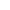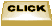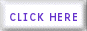Algebra THERE ARE THREE TYPES OF ALGEBRAIC ANSWERES TO EQUATIONS.ONE SOLUTION:     WHEN x IS EQUAL TO ONLY ONE ANSWER SUCH AS:     2x + 8 = 10   WHEN SOLVING YOU WILL FIND THAT X = 1NO SOLUTION:     WHEN X HAS NO SOLUTIONS     USUALLY THIS IS WHEN NEITHER SIDES OF THE EQUATION ARE EQUAL, THERE        IS NO VARIABLE LEFT AND THE CONSTANTS DO NOT EQUAL. SUCH AS :     2x + 8 = 2x - 9  IF YOU SUBTRACT 2X FROM BOTH SIDES YOU WILL BE LEFT               WITH 8 = -9 WHICH IS NOT TRUE/MULTIPLE OF INFINITE SOLUTIONS:     BOTH SIDES ARE EQUAL TO EACHOTHER.  FOR EXAMPLE     2X + 4 = 2(x + 2) DO CONVERTIONS SOLVE INEQUALITITES SOLVE EQUATIONSHOME